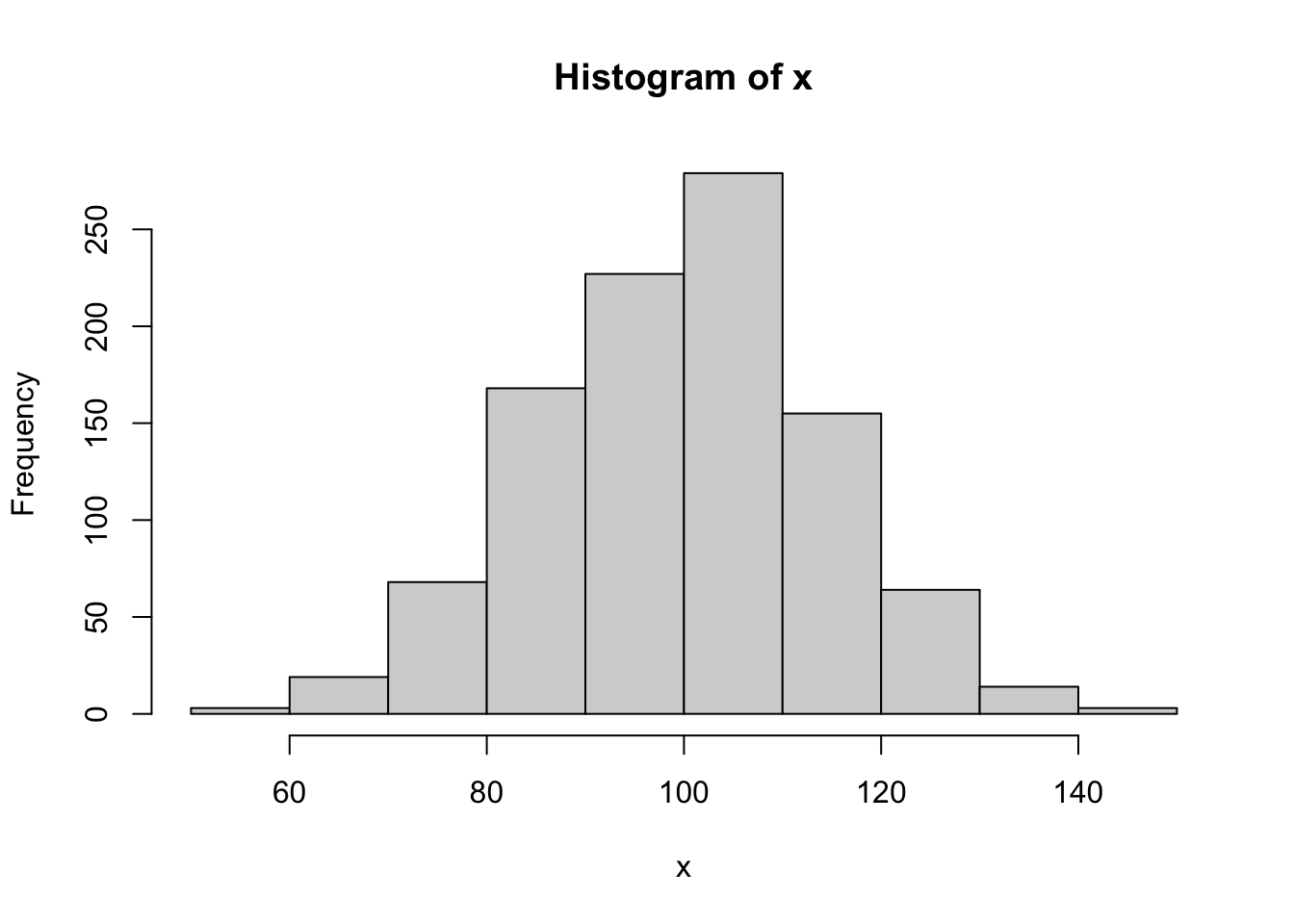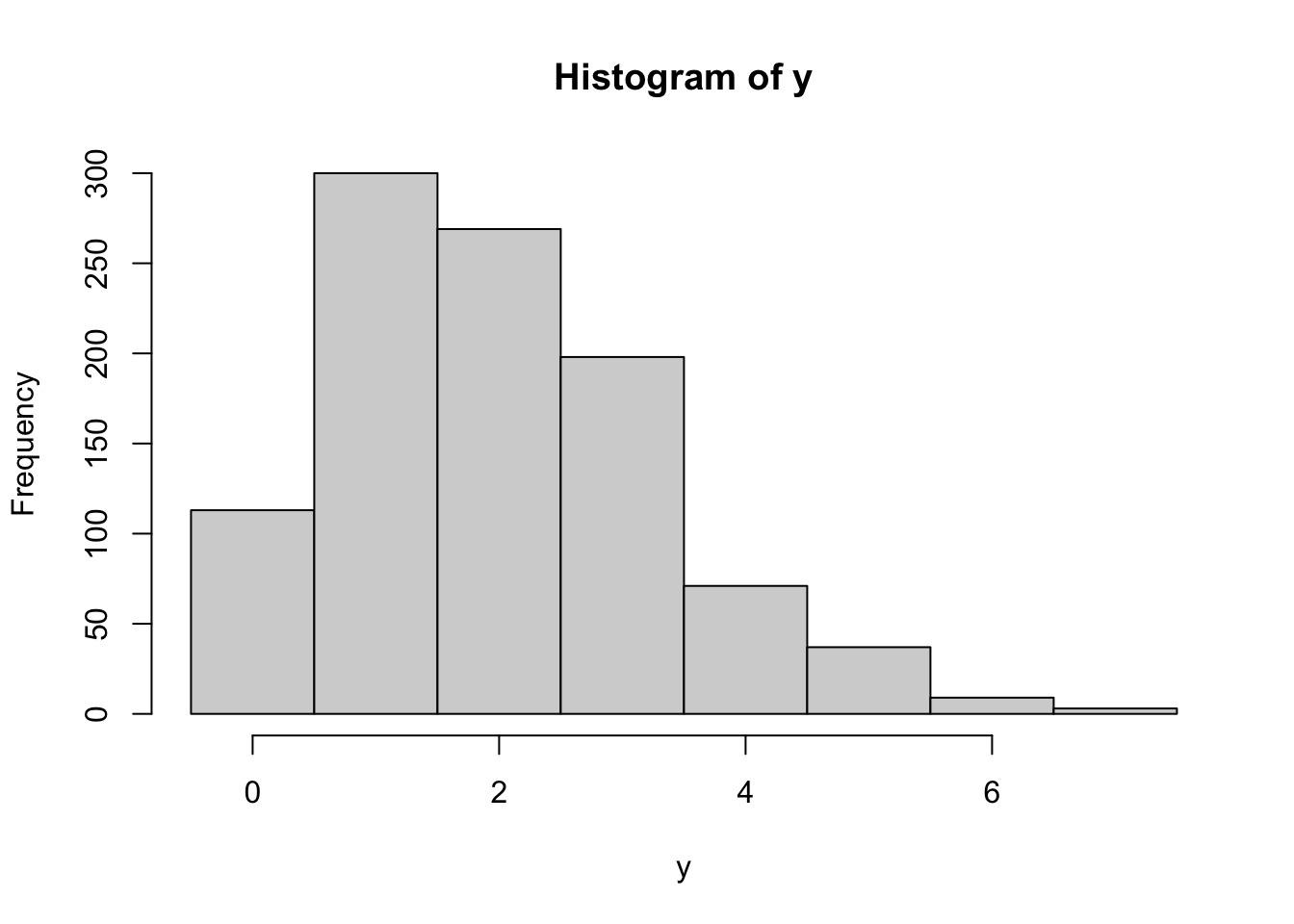The rand_bytes function binds to RAND_bytes in OpenSSL to generate cryptographically strong pseudo-random bytes. See the OpenSSL documentation for what this means.

rnd <- rand_bytes(10)
print(rnd)
  1d c5 e9 0e a6 53 c0 57 8f 63

Bytes are 8 bit and hence can have 2^8 = 256 possible values.

as.numeric(rnd)
   29 197 233  14 166  83 192  87 143  99

Each random byte can be decomposed into 8 random bits (booleans)

x <- rand_bytes(1)
as.logical(rawToBits(x))
 FALSE  TRUE  TRUE  TRUE  TRUE  TRUE FALSE FALSE

## Secure Random Numbers

rand_num is a simple (2 lines) wrapper to rand_bytes to generate random numbers (doubles) between 0 and 1.

rand_num(10)
  0.25480299 0.12251893 0.92505491 0.87976586 0.96091479 0.43481803
 0.72627878 0.16068469 0.42657462 0.09808706

To map random draws from [0,1] into a probability density, we can use a Cumulative Distribution Function. For example we can combine qnorm and rand_num to simulate rnorm:

# Secure rnorm
x <- qnorm(rand_num(1000), mean = 100, sd = 15)
hist(x)Same for discrete distributions:

# Secure rbinom
y <- qbinom(rand_num(1000), size = 20, prob = 0.1)
hist(y, breaks = -.5:(max(y)+1))